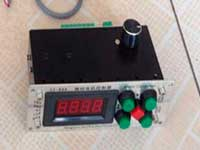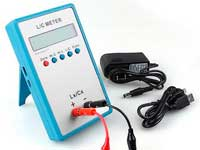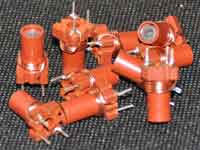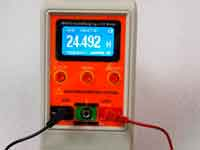Inductor with non circular winding - 4.0 out of 5 based on 1 vote
Rating 4.00 (1 Vote)

Inductor with noncircular windingQuite often, the inductor is wound on a coil-former having a square or polygonal shape. Such inductor can be represented as an equivalent cylindrical coil with the same winding length and number of turns. The easiest way is geometric. Either accept as the equivalent a cylindrical coil having an area of a cross section just the same as a polygon or with the same perimeter - the truth lies somewhere in the middle.

In 1946 F. Grover in the work "Calculation of inductance - working formulas and tables" leads tabular data of polygonal coil with matching to it cylindrical equivalent, that had been getting from experimental measurements. On the basis of these tables, R. Weaver in 2010-2012 derived an empirical formula that allows calculating the polygonal coil numerically.

Denote the radius of the circumcircle of the polygon as r0. It is obvious that 2r0 = D0. The cross-sectional area of the polygon is:And its perimeter:We denote the radius of the circle with length equal to the perimeter of the polygon as rP and the radius of the circle with the same area as the polygon -  rA, then it is obvious that rP = P/2π, and rA = √(A/π).

As you know,  the inductance of a infinitely long solenoid is proportional to its cross-sectional area. It would seem enough to determine rA, but it's not right for short coils. The equivalent radius rE = 2D can be found as the average value between rP and rA by introducing the correction factor kW, which varies from one when the length of the coil tends to zero and to zero - when the length of the coil goes to infinity. As a result, the equivalent winding radius of the coil is determined by the following formulae:where: l - winding length of the coil, and D0- diameter of a circle describing the polygon. The coefficient 368 selected empirically in order to the calculation had an accordance with F. Grover's tables.

The Coil32 uses this R. Weaver's numerical method. The error of calculation by this method is less than ±1.5% for the coils with a triangular cross-section of winding and becomes much smaller when you increase the number of sides of the coil.

Reference:

Сomments from anonymous guests are enabled with moderation.

Coil Winding MachineUS \$187.53
The system consists of individual components including a controller, a stepping motor, a foot switch, power adapter and output shaft. This design makes it very easy for you to integrate the system into your own project.

LC Meter LC200AUS \$37.99
Measuring Capacitance Range:
0.01 pF - 100mF
Measuring Inductance Range:
0.001 uH - 100H
Measurement accuracy:1%.

RF InductorsUS \$6.43
Variable Ferrite Core Inductor coil set 3.5Turns 25-100MHZ

Auto Ranging LCR MeterUS \$63.07
Meter can measure 0.00pF to 100.00mF capacitance, 0.000uH to 100.00H inductance and 0.00? to 10.000M? resistance. Very suitable for small value capacitor and inductor measurement.